# PHYS 170

Lecture 04a - Newton's Laws of Motion

## Introduction

Newton's Laws of Motion (the very brief version):

• velocity is either zero or constant when net force is zero.
• $$F=ma$$
• action = reaction, but in the opposite direction.

"Net force" means the total force you get after adding all the forces together as vectors. For example, if you have a $$5N$$-force pointing left, a $$2N$$-force pointing right, the net force is not $$7N$$, but $$3N$$ pointing left. Mathematically, the net force is found by: $$\vec{F_{net}} = (-5\hat i) + (2\hat i) = -3\hat i N$$ Never calculate the net force by summing up the magnitudes without taking the direction into account.

## The First Law

### Inertia and Mass

Newton's First Law is also known as the law of inertia.

Inertia is the tendency of an object to remain at rest or in motion with constant speed along a straight line.
Mass is a number that measures an object's inertia. In short, you can think "mass = inertia".

A moving ice puck moving on very smooth ice will keep moving because it has the tendency to remain in motion with constant speed, in other words because it has inertia.Image by Santeri Viinamäki [CC BY-SA 4.0], via Wikimedia Commons

### Mass vs Weight

In physics, mass and weight are not the same thing.

Difference Mass Weight
Meaning measures inertia, an intrinsic property that does not change gravitation force, value changes depeding on the strength of the gravitational field
Example you have the same mass on earth or on the moon you weigh less on the moon than on earth
Unit $$kg$$ $$N$$

The basic equation of weight is: $$F_{gravity} = mg$$ where $$g$$ is the acceleration due to gravity. Since weight is just the name for the force of gravity, we used the notation "$$F_{gravity}$$". Other common notations for weight are $$W, F_g$$, and in many cases we will simply write "$$mg$$" for weight. Also note how the unit matches on both sides because $$1N = 1kg m/s^2$$.

## The Second Law

The second law gives the relationship between force, mass and acceleration as $$F=ma$$ where $$F$$ is the net force on the mass.

### Try It Yourself (click to show)

The next few questions are based on the figure below of a mass being pushed by two forces: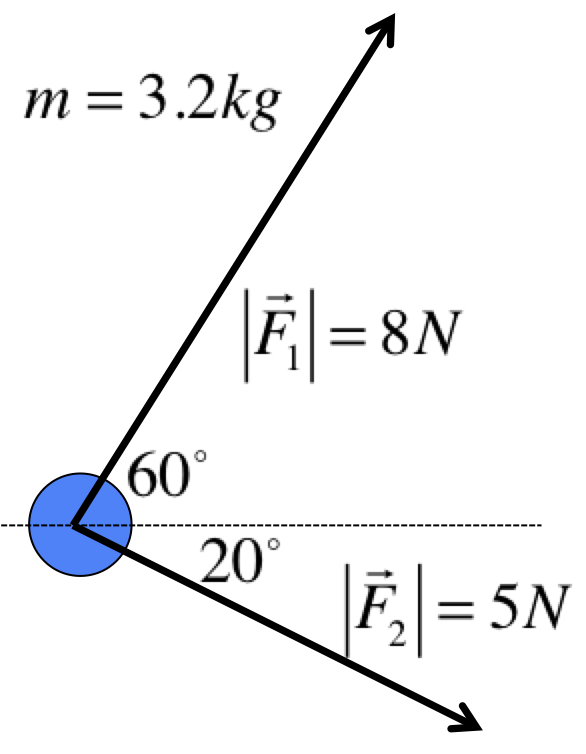Decompose based on the triangles below and be careful with the signs, remember South is negative. Go back to Web Lecture 01 for a refresher if you cannot remember how to do this.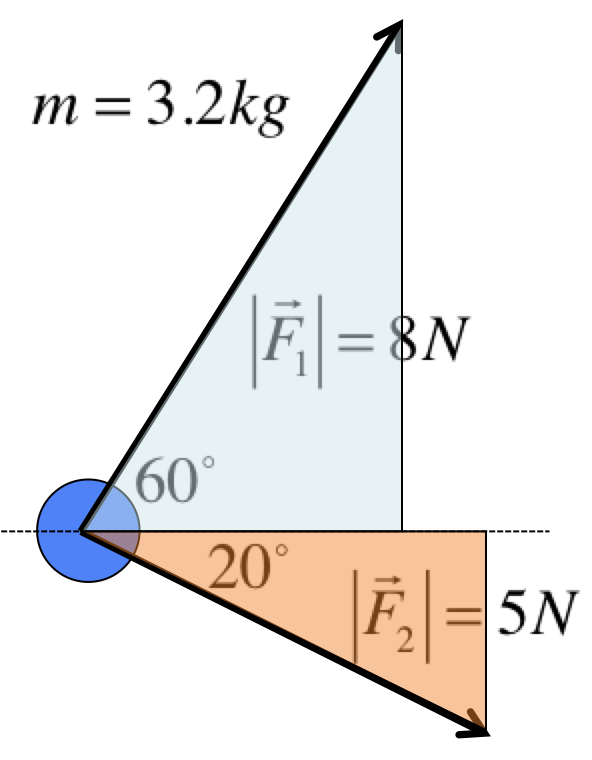$$\begin{eqnarray} \vec{F_1} &=& 8\cos 60^\circ \hat i + 8\sin 60^\circ \hat j = (4\hat i + 6.93\hat j)N \\ \vec{F_2} &=& 5\cos 20^\circ \hat i - 5\sin 20^\circ \hat j = (4.7\hat i - 1.71\hat j)N \end{eqnarray}$$
$$\begin{eqnarray} \vec{F_{net}} &=& m \vec{a} \\ \Rightarrow \vec{a} &=& \frac{1}{m}\vec{F_{net}} \\ &=& \frac{1}{3.2}(8.7\hat i + 5.22 \hat j) \\ &=& (2.72\hat i + 1.63\hat j)m/s^2 \\ \Rightarrow |\vec{a}| &=& \sqrt{2.72^2 + 1.63^2} = 3.17 m/s^2 \end{eqnarray}$$

## The Third Law

Newton's Third Law states that for every action (force), there is an equal and opposite reaction. In other words you cannot push without being pushed back just as hard. In a later chapter, you will see how the Third Law leads to the law of conservation of momemtum during collisions.

## Opposing Forces

Most of the problems you do will involve more than one forces, all point in different directions. It is essential that you know how to deal with this situation correctly. There are many methods to do this, but I will teach you one that is the easiest to apply to problems you see in this course.

Below shows the two possibilities you have to learn about:
(a)(b)These are called "free-body diagrams", or "force diagrams". Each is a dot with arrows coming out of it. The "dot" represents the mass, while each arrow is a force. On the outside, you could use another arrow to denote the acceleration.

In our convention, every variable on the force diagram represents only the magnitude, so they are all positive. We let the arrows represent the directions, and we do not use negative numbers, even if they point left or down. Do not use the "up is positive, down is negative" convention we had in chapter 1 and 2.

With this convention, this is how you apply the Second Law:

$$\begin{eqnarray} (\text{forces in the same direction as }a) - (\text{forces in the opposite direction to }a) &=& ma \\ \text{Or symbolically: } F_{same}-F_{opposite} &=& ma \end{eqnarray}$$
Again, the $$a$$ and the $$F$$ in this equation are magnitudes so they will all be positive numbers in this convention.(a) Since $$F_1$$ is opposite to $$a$$ and $$F_2$$ is in the same direction as $$a$$, we have: $$\begin{eqnarray} F_{2}-F_{1} &=& ma \\ \Rightarrow a &=& \frac{F_2-F_1}{m} \end{eqnarray}$$(b) Since $$F_1$$ is same direction as $$a$$ and $$F_2$$ is opposite to $$a$$, we have: $$\begin{eqnarray} F_{1}-F_{2} &=& ma \\ \Rightarrow a &=& \frac{F_1-F_2}{m} \end{eqnarray}$$
Be very careful with the order of the forces. If you reversed them you will get the wrong answer.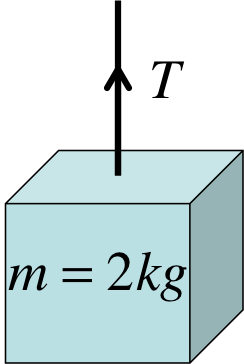A $$2kg$$ block is being accelerated upward at $$4m/s^2$$ by a cable. Find the tension $$T$$ in the cable.First you must start with a force diagram. There are two forces, the tension $$T$$ and the weight of the object $$mg$$. The question states the acceleration is upward so it is indicated in the diagram. $$\begin{eqnarray} F_{same}-F_{opposite} &=& ma \\ \Rightarrow T - mg &=& ma \\ \Rightarrow T &=& mg+ma = m(g+a) \\ &=& (2)(9.8 + 4) = 27.6N \end{eqnarray}$$ Note that tension is a force, so it is measured in $$N$$ just like all forces.

### Try It Yourself (click to show)First you must start with a force diagram. There are two forces, the tension $$T$$ and the weight of the object $$mg$$. The question states the acceleration is downward so it is indicated in the diagram. In this case, $$mg$$ is in the same direction as $$a$$ but $$T$$ is opposite. $$\begin{eqnarray} F_{same}-F_{opposite} &=& ma \\ \Rightarrow mg - T &=& ma \end{eqnarray}$$
$$\begin{eqnarray} mg - T &=& ma \\ \Rightarrow T &=& mg-ma = m(g-a) \\ &=& 2(9.8-4) = 11.6N \end{eqnarray}$$
If there is no acceleration, the two opposing forces must cancel out. This means they must have the same magnitude: $$\begin{eqnarray} T &=& mg \\ \Rightarrow T &=& 2(9.8) = 19.6N \end{eqnarray}$$

## Normal Force

"Normal Force" means perpendicular force. It usually occurs when an object is leaning on another solid object, such as the figure below. The normal force is always perpendicular to the surface producing it. Common notations are $$F_n, F_N$$, and $$n$$.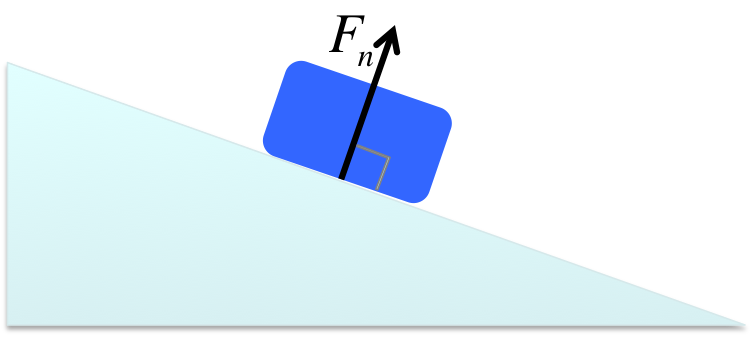One of the most common mistakes students make is to wrongly believe normal force is always $$mg$$, but as you will see in the examples below, it is not true in most cases.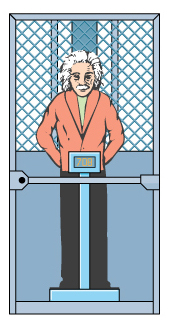Einstein ($$m=70kg$$) is on a scale in an elevator accelerating downward at $$5m/s^2$$. What is the reading of the scale?The scale actually measures the normal force $$F_N$$ on Einstein, not his weight $$mg$$. Because of this, the acceleration of the elevator will affect the reading. $$\begin{eqnarray} F_{same}-F_{opposite} &=& ma \\ \Rightarrow mg - F_N &=& ma \\ \Rightarrow F_N &=& mg - ma = m(g-a) \\ &=& 70(9.8-5) = 336N \end{eqnarray}$$ Note once again that we are using the convention where all the variables on the force diagrams are magnitudes, that is why even though $$a$$ points down we still substitue $$a = |\vec{a}|=+5m/s^2$$ in the equation above.

### Try It Yourself (click to show)First you must start with a force diagram. There are two forces, the normal force $$F_N$$ and the weight of Einstein $$mg$$. The question states the acceleration is upward so it is indicated in the diagram. In this case, $$F_N$$ is in the same direction as $$a$$ but $$mg$$ is opposite. $$\begin{eqnarray} F_{same}-F_{opposite} &=& ma \\ \Rightarrow F_N - mg &=& ma \end{eqnarray}$$
$$\begin{eqnarray} F_N - mg &=& ma \\ \Rightarrow F_N &=& mg+ma = m(g+a) \\ &=& 70(9.8+5) = 1036N \end{eqnarray}$$
If there is no acceleration, the two opposing forces must cancel out. This means they must have the same magnitude: $$\begin{eqnarray} F_N &=& mg \\ \Rightarrow F_N &=& 70(9.8) = 686N \end{eqnarray}$$$$\begin{eqnarray} mg - F_N &=& ma \\ \Rightarrow F_N &=& mg-ma = m(g-a) \\ \Rightarrow F_N &=& 70(9.8 - 9.5) = 21N \end{eqnarray}$$ The normal force is very small, meaning Einstein is barely in contact with the scale as the elevator is falling. If the acceleration of the elevator is exactly $$9.8m/s^2$$, the normal force would become zero and Einstein will feel he is floating in the elevator. This is what Einstein called "the happiest thought of his life".

## Multiple Forces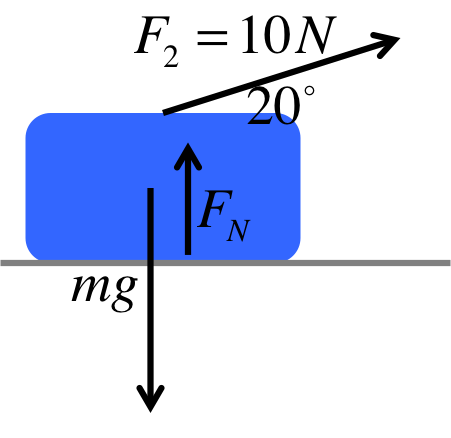First identify all the forces in the problem, as shown on the left.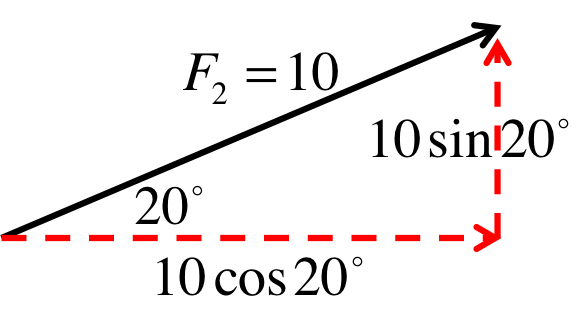Since the normal force $$F_N$$ is vertical, we need to identify all the vertical forces so we have to find the vertical component of $$F_2$$. We can ignore the horizontal component for this problem because vertical forces and horizontal ones do not affect each other.Draw only the vertical forces in a force diagram. Since the block has no vertical movement, the vertical acceleration $$a_y$$ is zero. This means all the vertical forces must cancel out: $$\begin{eqnarray} F_{up} &=& F_{down} \\ \Rightarrow F_N + 10\sin20^\circ &=& mg \\ \Rightarrow F_N &=& mg - 10\sin 20^\circ \\ &=& (2)(9.8) - 10\sin 20^\circ = 19.6 - 3.42 \\ &=& 16.18N \end{eqnarray}$$

### Simulation - Normal Force (click to hide)

Canvas not supported
Drag on the ground to tilt.
Drag on the blue arrow (the applied force) to change, or click "Remove Applied Force" to remove.
The red arrow represnts the total force.

### Try It Yourself (click to show)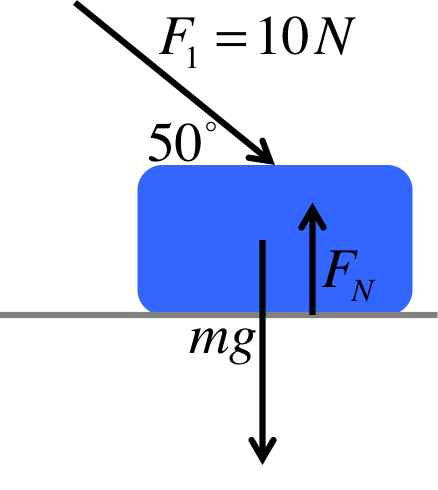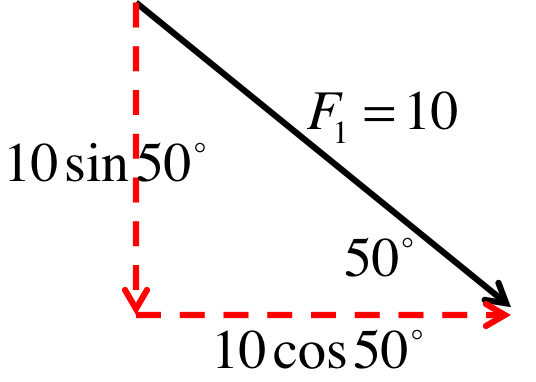The vertical component is the opposite side to $$50^\circ$$, so we use the $$\sin$$ function. We are going to use this in a force diagram so we will only need the magnitude (as stated in the question), which means we do not use the negative sign to track its downward direction.Draw only the vertical forces in a force diagram. Since the block has no vertical movement, the vertical acceleration $$a_y$$ is zero. This means all the vertical forces must cancel out: $$\begin{eqnarray} F_{up} &=& F_{down} \\ \Rightarrow F_N &=& mg + 10\sin 50^\circ \\ &=& (2)(9.8) + 10\sin 50^\circ = 19.6 + 7.66 \\ &=& 27.26N \end{eqnarray}$$

## Notations

$$\begin{eqnarray} W, F_g, F_{gravity}, mg &:& \text{weight}\\ F_n, F_N, n &:& \text{normal force}\\ \end{eqnarray}$$